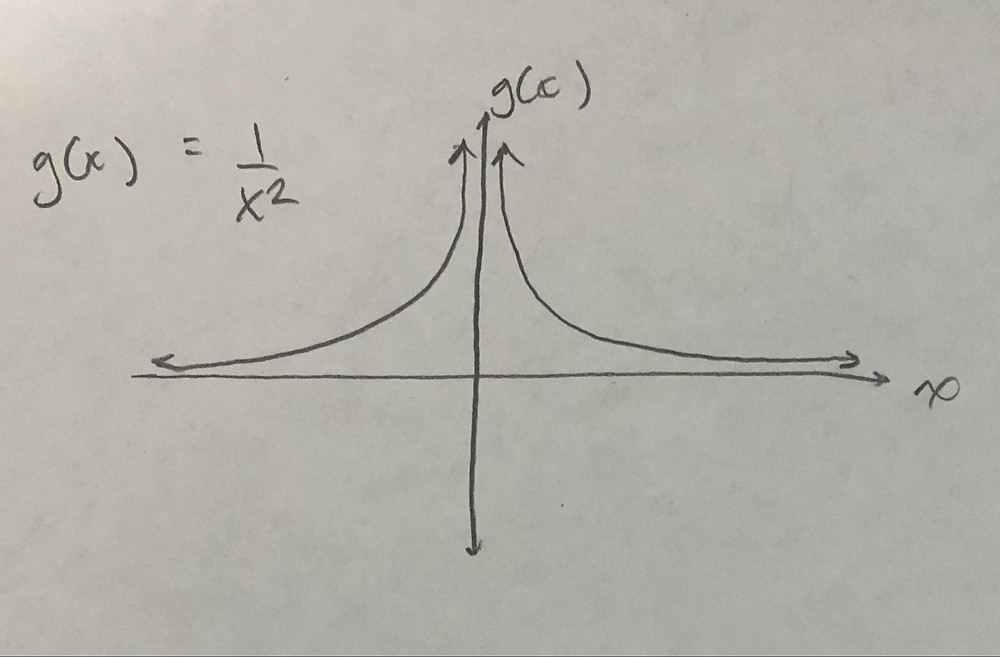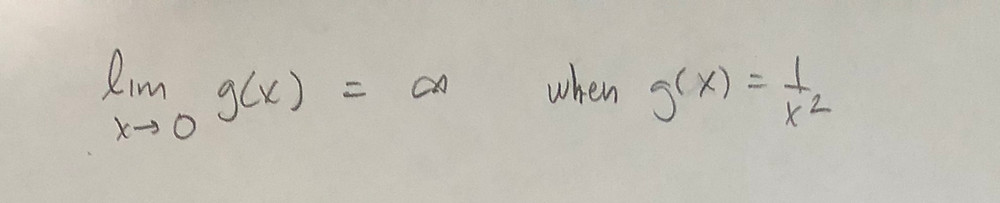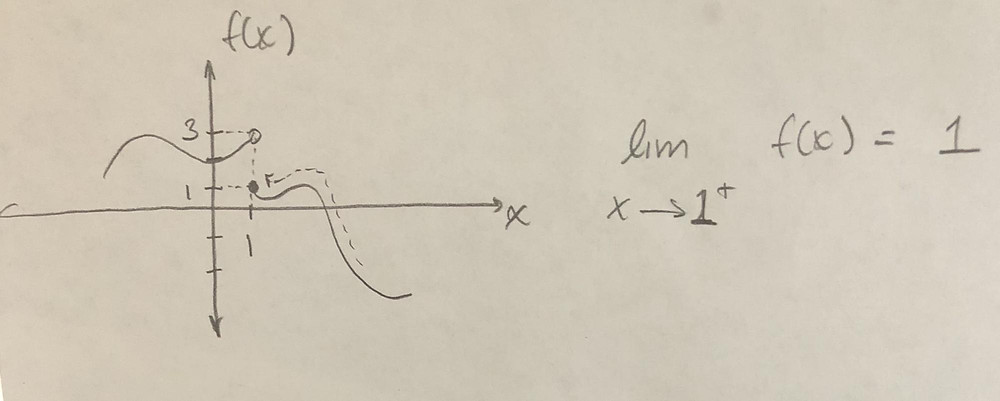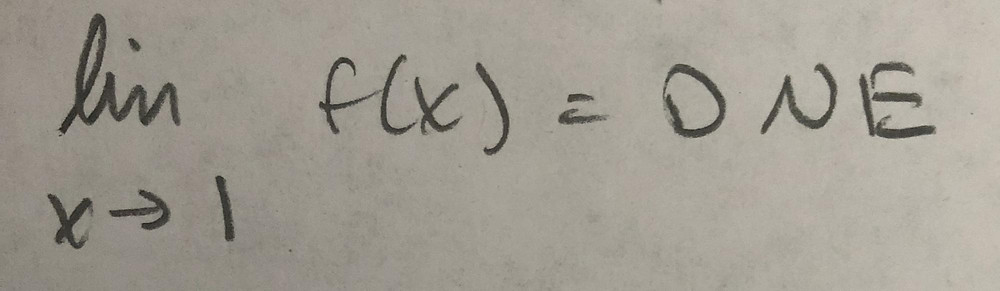# Infinity and DNE in Limits

Updated: Jan 11, 2020

The best way to approach why we use infinity instead of does not exist (DNE for short), even though they are technically the same thing, is to first define what infinity means.

Infinity is not a real number. It’s a mathematical concept meant to represent a really large value that can’t actually be reached. In terms of solutions of limits, it means that the equation you are taking the limit of will go in that direction forever.

For example:You have a vertical asymptote at the y-axis (which is x = 0), which means that the graph approaches, but never touches that axis. As you approach x = 0 from either the right or left, g(x) = 1/(x^2) will keep going higher and higher. You can imagine it going off the page and continuing upwards. In other words, the limit as x approaches zero of g(x) is infinity, because it keeps going up without stopping.So while infinity is technically meaningless/just a mathematical construct, it can be used to describe the outcome of limits when a function keeps going forever (which usually occurs when you have a vertical asymptote).

To be clear, the limit doesn’t actually exist, since we can’t assign a number to it (it goes on forever), so you could say:And that would be technically correct, but it’s more useful (and intuitive if you look at the graph) to say it goes to infinity. Likewise if you had:The limit as x approaches zero would be negative infinity, since the graph goes down forever as you approach zero from either side:As a general rule, when you are taking a limit and the denominator equals zero, the limit will go to infinity or negative infinity (depending on the sign of the function).

So when would you put that a limit does not exist?

When the one sided limits do not equal each other.

First, a one sided limit is when you approach a value from a single side. You specify which side you are coming from with a plus or minus over what x is approaching. If you approach from the right, you use +, from the left you use -.

For example:As you approach x = 5 from the right (indicated by the dotted line), f(x) = 2. This is a one sided limit coming from the right side.As you approach x = 5 from the left, f(x) = 2 again. This is a one sided limit coming from the left.

Since the limits from both the right and left are the same. The limit exists and is equal to what both of the one sided limits are equal to.

So:The one sided limits are not equal, so the limit as x approaches 1 does not exist.As a side note, positive and negative infinity can be what x is approaching. So:This has mathematical significance and is frequently used:This one means nothing/isn’t a valid statement.

With limits approaching infinity, if infinity ends up in the denominator, then the limit normally equals 0

If you end up with infinity in the numerator and denominator, then you have to see if the highest degree (the one with the biggest exponent) of x is in the numerator or denominator.## Shayan Raofi

January 11, 2020

View Profile

#### Get in Touch

Thanks! We'll reach out shortly.